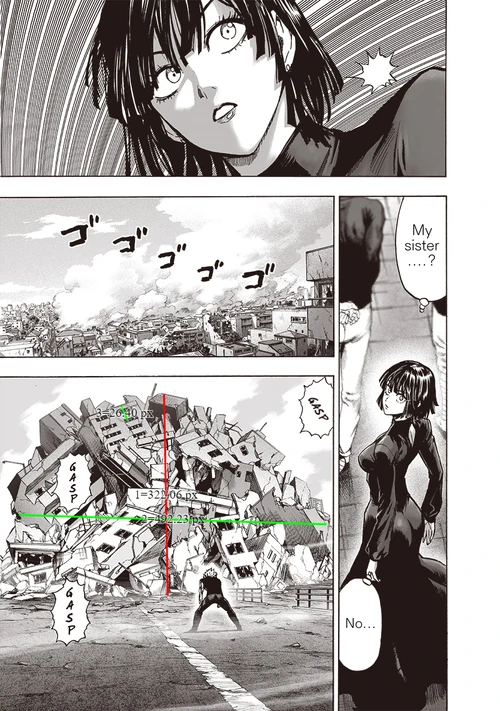## FANDOM

24,851 Pages

​​​​​In this feat, Gearsper destroys some buildings, turning them in spikey projectiles and shoots them at Nyan. I've updated this calc.

I'll assume Nyan is 1.85 meters since he looks kinda tall when compared to other humans, who must be 1.7m since that's the average.
If 1.8m = 88.8p, then 1p = 0.02083 meters. He's, close to a building which stands at 587.7p tall, or 12.24 meters tall. The average height for 1 floor is that of 3m, and we clearly see 4 floors so it makes sense here.

" Rules of thumb around the construction industry are 200 pounds per square foot for a single-level home, 275 for two levels and 350 for three levels. "
By logic, we add in an extra 75 pounds per square foot since this is a 4 floors tall building. For a total of 425 pounds per square foot, or 192.77kg per 0.092903 square meters, or 2075kgs per square meter. The area of the roof seems to be that of [(130.65*0.02083)*(240.3*0.02083)] square meters, for a total of 13.62 square meters per floor, so it's a 54.48m^2 building. Multiplying that to the 2075kgs we get 113046kgs, or 113.046 tons.

The fragmentation of RC is 28 j/cc.

The density of RC is 2400kg/cubic meter. If said building weights 113 tons, then it has a volume of about 47 cubic meters or 47000000 cc.

Resulting in 47000000*28 = 1318240000J or 0.31 tons. The fact he did this to 7 buildings gets him to '2.17' tons or High 8-C.In this other panel, we can see the aftermatch of the buildings he destroyed. Average height for a floor is 3m, so 1p = 0.113m.

The building debris seems to have a ellipsoid form, so i'll be calc'ing the volume of it.

321p = 36.27m. 36.27/2 = 18.13m. (I used this as depth as well)

492p = 55.59m. 55.59/2 = 27.8m.

For a total of 38276.21 cubic meters. This would end up being 3827621000000cc. With the fragmentation values for RE, it would end up in 25.61 kilotons, 7-C.

I don't think this result should be used because:

a) It assumes the ellipse to be made of pure RC, which it isn't.

​​​​​​b) It's outlierish.

Now for the 3rd scan. This is where things get really messy since i have little to no idea on how to calc this, but i'll give it a try.

Again, Nyan should be around 1.85m.
I'll be using one of the spikes that are close to him as an average, since there are both small and big spikes evenly.

All of the spikes have a cone shape, so i'll be attempting to calc (and failing miserably) the energy needed to do that kind of compression.

The volume of one spike is equal to 1.8 m^3, using one of them as average.

This states: "The actual comprehensive strength of various concrete mixtures varies from 2,500 psi to as much as 10,000 psi".

The aritmethic medium being 3750psi or 25855350 Pa or 25.85 Mpa.

Since there are around 25 spikes excluding the ones on the background, i'll do a low, a mid, and a high end using, respectively 2500, 3750 and 10000psi.

Low-End:  17.23689 * 18000000 * 25 = 7756600500J or 1.85 tons.

Mid-End: 25.85 * 18000000 * 25 = 11632500000J or 2.78 tons.

High-End: 69 * 18000000 * 25 = 31050000000J or 7.42 tons.

If we were to calc the KE of said spikes, even the furtherst ones, on the background, hitted Nyan in the timeframe of 1 panel. Of course that has no definitive timeframe, but i, and most, usually interpret it as an instant of about 0.1 sec. The closest spike in the 1st perspective layer is 10.6 meters away from Nyan, i tried to calc the ones in the background that seem to be even further away but it ended up in unrealistic results such as 5 meters.

​​​​​​

​​​​
​​​Assuming the spikes moved 10.6 meters in 0.1s we get a speed of 106m/s.

KE would, then, be 0.5 * 791322 * 106 * 106 = 1.38 tons.

Community content is available under CC-BY-SA unless otherwise noted.